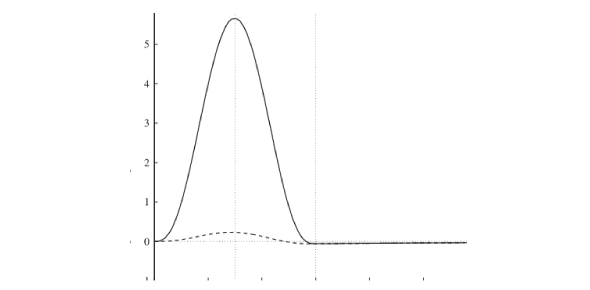What is the best way to calculate the magnitude of a force? - ProProfs DiscussTopicsMoreProductsMore+ Ask Question

# What is the best way to calculate the magnitude of a force?Change Image    Delete

This question is part of Distance and Displacement Quiz
Asked by Bilbojaggens, Last updated: Jun 29, 2020

###Request 0FollowShareAnswer AnonymouslyAnswer LaterCopy Link#### c.Dorothy

C.Dorothy

To measure the force of something, you must first learn the formula for force. It is force which is equal to the mass times the acceleration. The mass should be written in kilograms. If the mass is not written in kilograms, then it should be converted. These are called the International System of Units. Now plug in the mass number and the acceleration number. Then multiply those two numbers together.

If you have the mass and force and need to find the acceleration, then that can be done too. You will divide the force by the mass to get the acceleration. There are differences between weight, force and mass. Weight is only a force when the force is caused by a gravitational object.Qqswadwfe2 ed wsd sqad sqdq dwq dsa qsd qdwdqa qwd q qsd qwdd q dexplain how can it end in a decimal when the distance was not in decimals1.4km#### John Smith

John SmithCalculating the force, or the "resultant vector," requires the ever-useful Pythagorean theorem.Search for Google imagesSelect a recommended image
Upload from your computerCancelSearch for Google imagesSelect a recommended image
Upload from your computerCancelSearch for Google imagesSelect a recommended image
Upload from your computerCancel